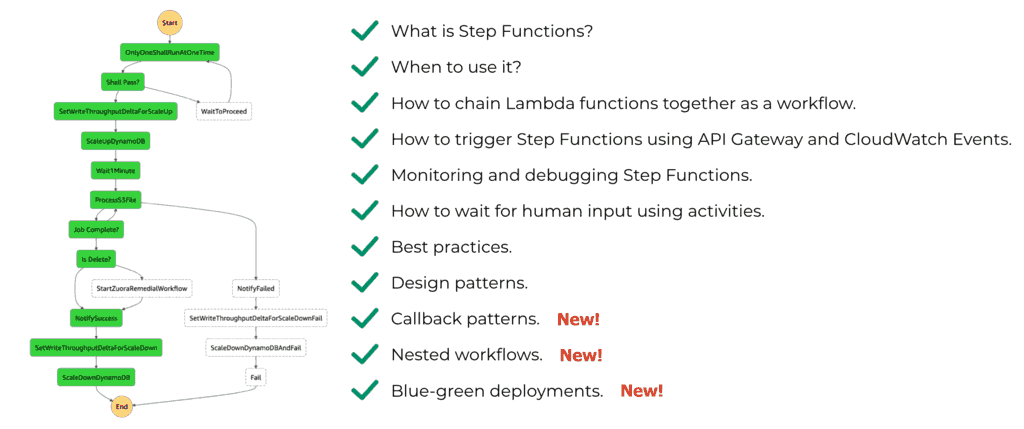# Project Euler – Problem 87 Solution

#### Problem

The smallest number expressible as the sum of a prime square, prime cube, and prime fourth power is 28. In fact, there are exactly four numbers below fifty that can be expressed in such a way:

28 = 22 + 23 + 24

33 = 32 + 23 + 24

49 = 52 + 23 + 24

47 = 22 + 33 + 24

How many numbers below fifty million can be expressed as the sum of a prime square, prime cube, and prime fourth power?

#### Solution

```// generate all prime numbers under <= this max
let max = int64(sqrt(double(50000000L)))

// initialise the list with 2 which is the only even number in the sequence

// only check the prime numbers which are <= the square root of the number n
let hasDivisor n =
|> Seq.takeWhile (fun n' -> n' <= int64(sqrt(double(n))))
|> Seq.exists (fun n' -> n % n' = 0L)

// only check odd numbers <= max
let potentialPrimes = Seq.unfold (fun n -> if n > max then None else Some(n, n+2L)) 3L

// populate the prime numbers list
for n in potentialPrimes do if not(hasDivisor n) then primeNumbers <- primeNumbers @ &#91;n&#93;

// use the same hasDivisor function instead of the prime numbers list as it offers
// far greater coverage as the number n is square rooted so this function can
// provide a valid test up to max*max
let isPrime n = if n = 1L then false else not(hasDivisor(n))

|> Seq.collect (fun n ->
|> Seq.map (fun n' -> pown n 2 + pown n' 3)
|> Seq.takeWhile (fun sum -> sum < 50000000L))
|> Seq.collect (fun sum ->
|> Seq.map (fun n -> sum + pown n 4)
|> Seq.takeWhile (fun sum' -> sum' < 50000000L))
|> Seq.distinct
|> Seq.length
```

The biggest prime that can appear in the equation is equals to the square root of 50000000 – 8 – 16, which, incidentally is just over 7000, so the numbers of primes involved is reasonably small which bodes well for a fast solution!

The logic in this solution is otherwise simple, using the cached prime numbers list to first generate numbers (< 50 million) that can be written as the sum of a prime square and prime cube; then for each number see if we can add a prime fourth power and get a sum less than 50 million.

One thing that caught me out initially was the need to search for distinct numbers as some of these numbers do overlap, but otherwise this is a solution which runs happily under 3 seconds.Hi, I’m Yan. I’m an AWS Serverless Hero and the author of Production-Ready Serverless.

I specialise in rapidly transitioning teams to serverless and building production-ready services on AWS.

Are you struggling with serverless or need guidance on best practices? Do you want someone to review your architecture and help you avoid costly mistakes down the line? Whatever the case, I’m here to help.Check out my new course, Complete Guide to AWS Step Functions. In this course, we’ll cover everything you need to know to use AWS Step Functions service effectively. Including basic concepts, HTTP and event triggers, activities, callbacks, nested workflows, design patterns and best practices.